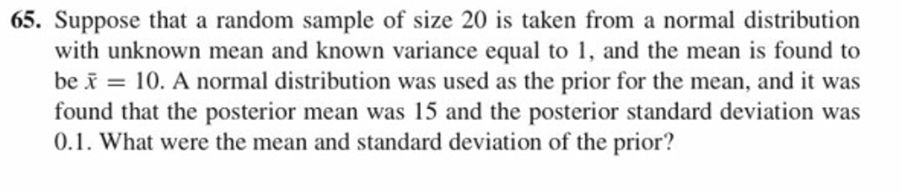65. Suppose that a random sample of size 20 is taken from a normal distributionwith unknown mean and known variance equal to 1, and the mean is found tobe x = 10, A normal distribution was used as the prior for the mean, and it wasfound that the posterior mean was 15 and the posterior standard deviation wa:s0.1. What were the mean and standard deviation of the prior?

Questionhelp_outlineImage Transcriptionclose65. Suppose that a random sample of size 20 is taken from a normal distribution with unknown mean and known variance equal to 1, and the mean is found to be x = 10, A normal distribution was used as the prior for the mean, and it was found that the posterior mean was 15 and the posterior standard deviation wa:s 0.1. What were the mean and standard deviation of the prior? fullscreen
Step 1

Introduction:

Suppose X1, X2, …, Xn be a random sample of size n taken from a normal distribution with mean μ and variance σ2, that is, Xi ~ N (μ, σ2) for all i from 1 to n, where σ2 is known. The mean, μ is a random variable with prior normal distribution, such that μ ~ N (μ­0, τ02).

Then, the posterior distribution of μ based on the observed values x1, x2, …, xn of X1, X2, …, Xn respectively is normally distributed, such that  that μ|x ~ N (μ­n, τn2), where:

Step 2

Calculation:

Here,

Step 3

Calculation continued:...

Want to see the full answer?

See Solution

Want to see this answer and more?

Our solutions are written by experts, many with advanced degrees, and available 24/7

See Solution
Tagged in

Other